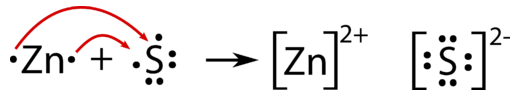# 5.5: Redox Reactions and Ionic Compounds

$$\newcommand{\vecs}{\overset { \rightharpoonup} {\mathbf{#1}} }$$ $$\newcommand{\vecd}{\overset{-\!-\!\rightharpoonup}{\vphantom{a}\smash {#1}}}$$$$\newcommand{\id}{\mathrm{id}}$$ $$\newcommand{\Span}{\mathrm{span}}$$ $$\newcommand{\kernel}{\mathrm{null}\,}$$ $$\newcommand{\range}{\mathrm{range}\,}$$ $$\newcommand{\RealPart}{\mathrm{Re}}$$ $$\newcommand{\ImaginaryPart}{\mathrm{Im}}$$ $$\newcommand{\Argument}{\mathrm{Arg}}$$ $$\newcommand{\norm}{\| #1 \|}$$ $$\newcommand{\inner}{\langle #1, #2 \rangle}$$ $$\newcommand{\Span}{\mathrm{span}}$$ $$\newcommand{\id}{\mathrm{id}}$$ $$\newcommand{\Span}{\mathrm{span}}$$ $$\newcommand{\kernel}{\mathrm{null}\,}$$ $$\newcommand{\range}{\mathrm{range}\,}$$ $$\newcommand{\RealPart}{\mathrm{Re}}$$ $$\newcommand{\ImaginaryPart}{\mathrm{Im}}$$ $$\newcommand{\Argument}{\mathrm{Arg}}$$ $$\newcommand{\norm}{\| #1 \|}$$ $$\newcommand{\inner}{\langle #1, #2 \rangle}$$ $$\newcommand{\Span}{\mathrm{span}}$$$$\newcommand{\AA}{\unicode[.8,0]{x212B}}$$

Nitric acid has many uses in the manufacture of fertilizers and explosives. Most nitric acid is manufactured from ammonia using a three-step process. The ammonia is oxidized to $$\ce{HNO_3}$$ through the formation of several nitrogen oxides, finally resulting in the acid.

## Redox Reactions and Ionic Compounds

In the course of a chemical reaction between a metal and a nonmetal, electrons are transferred from the metal atoms to the nonmetal atoms. For example, when zinc metal is mixed with sulfur and heated, the compound zinc sulfide is produced. Two valence electrons from each zinc atom are transferred to each sulfur atom.Figure $$\PageIndex{1}$$: Reaction between zinc and sulfur.

Since the zinc is losing electrons in the reaction, it is being oxidized. The sulfur is gaining electrons and is thus being reduced. An oxidation-reduction reaction is a reaction that involves the full or partial transfer of electrons from one reactant to another. Oxidation is the full or partial loss of electrons or the gain of oxygen. Reduction is the full or partial gain of electrons or the loss of oxygen. A redox reaction is another term for an oxidation-reduction reaction.

Each of these processes can be shown in a separate equation called a half-reaction. A half-reaction is an equation that shows either the oxidation or the reduction reaction that occurs during a redox reaction.

\begin{align*} &\text{Oxidation:} \: \ce{Zn} \rightarrow \ce{Zn^{2+}} + 2 \ce{e^-} \\ &\text{Reduction:} \: \ce{S} + 2 \ce{e^-} \rightarrow \ce{S^{2-}} \end{align*}\nonumber

It is important to remember that the two half-reactions occur simultaneously. The resulting ions that are formed are then attracted to one another in an ionic bond.

Another example of an oxidation-reduction reaction involving electron transfer is the well-known combination of metallic sodium and chlorine gas to form sodium chloride:

$2 \ce{Na} + \ce{Cl_2} \rightarrow 2 \ce{NaCl}\nonumber$

The half reactions are as follows:

\begin{align*} &\text{Oxidation:} \: \ce{Na} \rightarrow \ce{Na^+} + \ce{e^-} \\ &\text{Reduction:} \: \ce{Cl} + \ce{e^-} \rightarrow \ce{Cl^-} \end{align*}\nonumber

We will concern ourselves with the balancing of these equations at another time.

## Summary

• An oxidation-reduction reaction, or redox reaction, is a reaction that involves the full or partial transfer of electrons from one reactant to another.
• Oxidation is the full or partial loss of electrons or the gain of oxygen.
• Reduction is the full or partial gain of electrons or the loss of oxygen.
• A half-reaction is an equation that shows either the oxidation or the reduction reaction that occurs during a redox reaction.
• Examples of oxidation-reduction reactions are shown.

5.5: Redox Reactions and Ionic Compounds is shared under a CC BY-NC license and was authored, remixed, and/or curated by LibreTexts.